+
Isolating Variables
Solving Linear Equations and Inequalities
0
of 0 possible points

# Isolating Variables

Author: Sophia Tutorial
##### Description:

Identify the operations needed to isolate a variable in an equation.

(more)

Sophia’s self-paced online courses are a great way to save time and money as you earn credits eligible for transfer to many different colleges and universities.*

No credit card required

37 Sophia partners guarantee credit transfer.

299 Institutions have accepted or given pre-approval for credit transfer.

* The American Council on Education's College Credit Recommendation Service (ACE Credit®) has evaluated and recommended college credit for 33 of Sophia’s online courses. Many different colleges and universities consider ACE CREDIT recommendations in determining the applicability to their course and degree programs.

Tutorial
what's covered
1. Process of Solving an Equation
2. Review of Inverse Operations
3. Applying Inverse Operations
4. Simplifying Before Isolating a Variable

# 1. Process of Solving an Equation

When solving an equation for a variable, our main goal is to isolate a variable. In other words, we want to get the variable by itself on one side of the equation, with all other expressions on the other side of the equals sign. In this process, we must always remember that if we perform an operation on one side of the equal sing, we must do the same on the other side of the equal sign. Let's look at an example: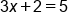Solve for x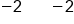Subtract 2 from both sides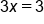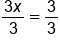Divide both sides by 3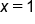Our Solution

big idea
Whatever we do on one side of the equation has to be done on the other side of the equation. This is known as the Rule of Equality.

# 2. Review of Inverse Operations

When isolating a variable, we need to keep the following in mind:

Operation Inverse Operation
Multiplication Division
Division Multiplication
Powers Roots
Roots Powers

# 3. Applying Inverse Operations

A good rule of thumb is to isolate the outermost operations surrounding the variable first, working our way inwards until we isolate the variable. Let's look at an example: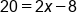Solve for x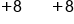Add 8 to both sides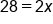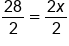Divide both sides by 2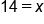Our Solution

hint
In general, we apply the inverse operations following the reverse order of operations to isolate a variable.

# 4. Simplifying Before Isolating a Variable

Sometimes when we try to isolate a variable, it may be better to simplify the equation before we perform any inverse operations. This is illustrated below: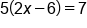Solve for x

There are two ways we can go about solving this equation. First, we can distribute 5 into the 2x and –6, and then isolate the variable, or we can divide both sides of the equation by 5 first, and then solve for x. Either method is value, and you are free to use either when trying to isolate the variable. Let's take a look at how we can use both methods to solve the equation above:

By distribution:Solve for x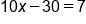Distribute 5 into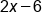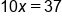Add 30 to both sides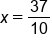Divide both sides by 10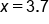Our Solution

Dividing 5 first:Solve for x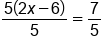Divide both sides by 5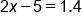Express 7/5 as a decimal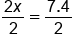Divide both sides by 2Our Solution

Let's look at another example where combining like terms before attempting to isolate the variable can be helpful: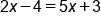Solve for x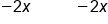Move the x terms to one side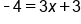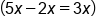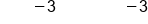Move constant term to one side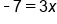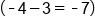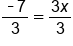Divide both sides by 3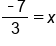Our Solution

hint
When trying to isolate a variable, it is always a good idea to simplify the equation as much as possible before starting to isolate the variable with inverse operations. This usually means that we should combine like terms whenever possible.
summary
The process of solving an equation involves isolating the variable you want to solve for. When isolating a variable, it is helpful to have a review of inverse operations: addition and subtraction are inverse, multiplication and division are inverse and powers and roots are inverse. Keep in mind when applying inverse operation that this will cancel the operations around the variable. Also, in using the inverse operations, use the order of operations in reverse order. Finally, simplifying before isolating a variable, such as distributing or combining like-terms, can be helpful.
Rating